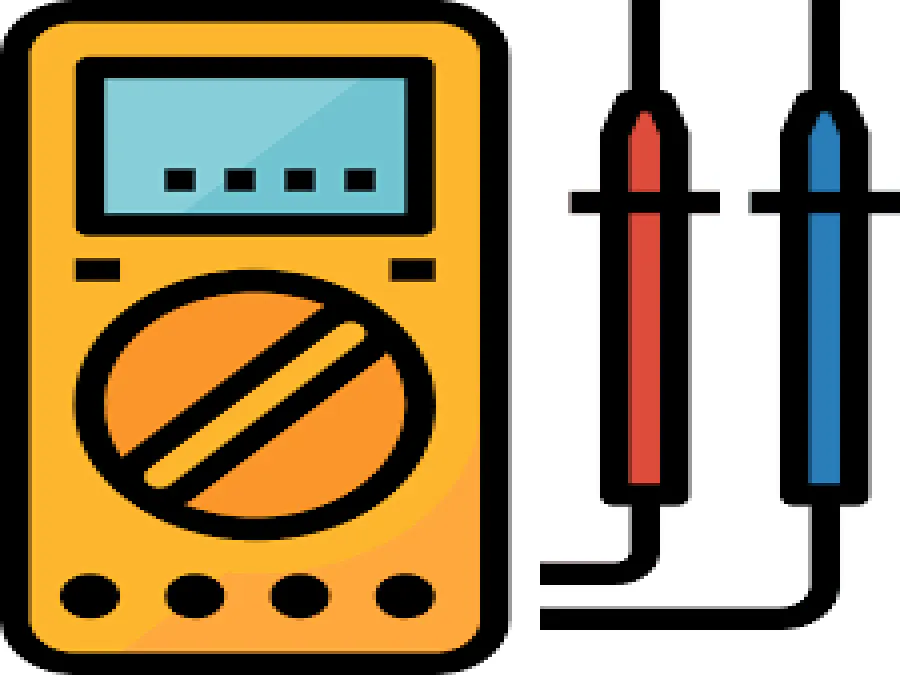Project tutorial# Ohm meter with Arduino Uno © LGPL

Measure and display an unknown resistance using Arduino uno

• 4,660 views
• 0 comments
• 11 respects

## About this project

In this project we measure an unknown resistance using Arduino Uno and a simple voltage divider circuit.

### The voltage divider

With voltage divider we can take a portion of input voltage as output. This is achieved by connecting two resistances in series connection. Because they are connected in series, the same current I runs through them:

If we solve this equation for Z2 we have:

If all values apart from Z2 are known, we can compute the unknown resistance using Vout.

### Resistors with large values

This setup is effective as long as Z1 is close to Z2. The readings are not accurate for large values of Z2 compared to Z1:

## Code

##### Untitled fileC/C++
```/*
The program reads the analog values of A0. These values are in the range of 0-1023.
*/

#include <LiquidCrystal_I2C.h>
#include <LCD.h>

#define analogInput A0
#define R1 220
#define vin 5
#define BACKLIGHT_PIN 3
#define En_pin             2
#define Rw_pin             1
#define Rs_pin             0
#define D4_pin             4
#define D5_pin             5
#define D6_pin             6
#define D7_pin             7

LiquidCrystal_I2C lcd(0x27, En_pin,Rw_pin,Rs_pin,D4_pin,D5_pin,D6_pin,D7_pin);

void setup() {

Serial.begin(9600);

}

void loop() {
int sensorValue=analogRead(analogInput);
float voltage=sensorValue*(5.0/1023.0);
screen(String(resistance(voltage)));
delay(3000);
}

int resistance(float vout){
return R1*(1/(vin/vout-1));
}

void screen(String text){
//Define the LCD as 16 column by 2 rows
lcd.begin (16,2);

//Switch on the backlight
lcd.setBacklightPin(BACKLIGHT_PIN,POSITIVE);
lcd.setBacklight(HIGH);

//goto first column (column 0) and first line (Line 0)
lcd.setCursor(0,0);

//Print at cursor Location
lcd.print("Resistor value is: ");

//goto second column and first line
lcd.setCursor(0,1);
lcd.print(text);
lcd.print(" ohm");

}
```

## Schematics

ohmmeter_sketch_Hj4WcaOhom.fzz

## Comments

#### Arduino Ohm Meter

Project showcase by lucian_vdo

• 7,588 views
• 5 comments
• 12 respects

#### Simple Arduino Uno - ESP 8266 Integration

Project tutorial by circuito.io team

• 117,561 views
• 25 comments
• 97 respects

#### Control Arduino Uno Using ESP8266 WiFi Module and Blynk App

Project tutorial by Adithya TG

• 51,021 views
• 14 comments
• 48 respects

#### Multicolor Knight Rider with RGB PL9823 LED + Arduino UNO

Project tutorial by Giovanni Gentile

• 6,840 views
• 0 comments
• 16 respects

#### Multifunctional Watch With Arduino Uno

Project showcase by shaqibmusa94

• 4,763 views
• 2 comments
• 9 respects

#### Arduino UNO and Vibration Sensor with Relay

Project tutorial by MichDragstar

• 2,747 views
• 2 comments
• 10 respects